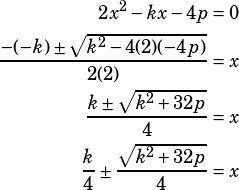Can you explain #15 in Section 3 of test 4? I understand how to do the problem but was wondering if there is a shortcut or trick to seeing the correct answer?

Great question! First, I don’t know if this is really a shortcut but for me this question becomes much easier to tackle when I multiply by 2 to eliminate the fraction. So the quadratic I’m working with after multiplying and rearranging is:So, for quadratic formula purposes,,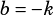, and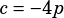.

The real shortcut, for this question and ones like it, is to focus on the differences in the answer choices rather than doing the whole quadratic formula process and trying to match a choice.

In this question, for example, you might notice that you only have two choices for the first term:or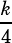. If you start by focusing only on that term, you can eliminate half the choices right away!

The quadratic formula is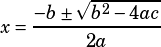. That means the first term is. In this question, then, the first term is. So we know the answer must be A or B.

From there, we know the bit under the radical must either be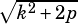or.

Since the radical bit equals, we can plainly see thatis the way to go. Choice B is the answer.

The full algebra for anyone who came here looking for it: